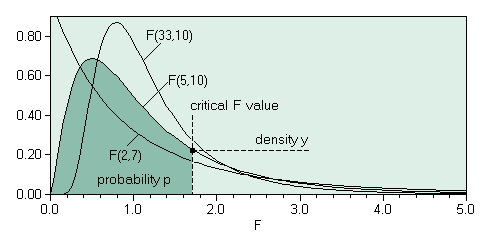The SDL Component Suite is an industry leading collection of components supporting scientific and engineering computing. Please visit the SDL Web site for more information....## FDistriQuantileUnit: SDL_statis Class: none Declaration: function FDistriQuantile (p: Double; df1, df2: integer): double;

The function FDistriQuantile returns the value of the quantile of the F distribution for a given probability p, and a specified number of degrees of freedom df1 and df2. The parameter p may take any value in the open interval (0,1). The parameters df1, and df2 may take values between 1 and MaxInt. If FDistriQuantile is called using invalid arguments a value of 0.0 is returned.Hint 1: The calculated value is exact to about 8 significant figures.

 Hint 2: The function FDistriQuantile calculates the quantile by using the integral from 0.0 to the F value. This differs from the convention of how the integral of the F distribution is used in many statistical packages, which tabulate the integral between +infinity and the F value. Thus you have to use 1-p to get comparable results. For example, in order to calculate the function FINV(p,df1,df2) of Microsofts Excel® you have to use FDistriQuantile(1-p,df1,df2).

Last Update: 2020-Aug-12